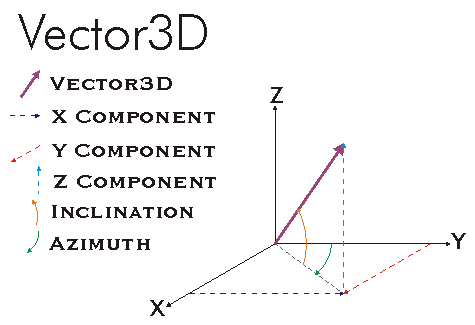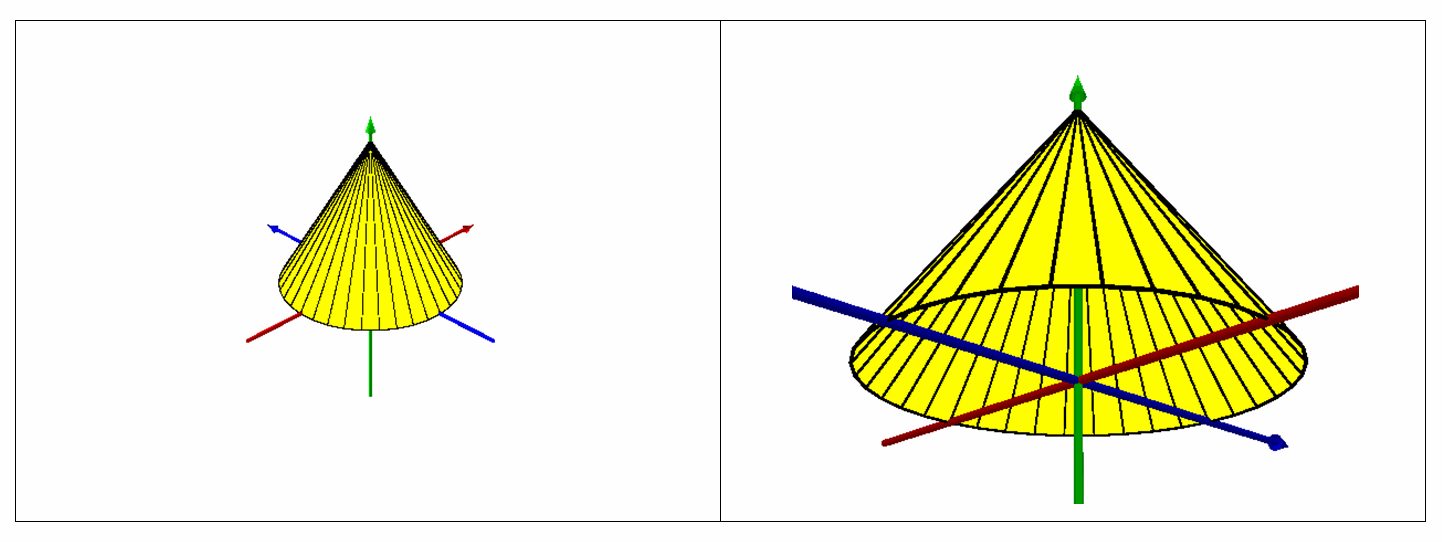This document is archived and information here might be outdated.  Recommended version.

IVector3D Interface (ArcObjects .NET 10.8 SDK)
 ArcObjects Help for .NET developers > ArcObjects Help for .NET developers > ArcObjects namespaces > Geometry > ESRI.ArcGIS.Geometry > Interfaces > IV > IVector3D Interface
 ArcGIS Developer Help

# IVector3D Interface

#### Product Availability

Available with ArcGIS Engine, ArcGIS Desktop, and ArcGIS Server.

#### Description

A 3 Dimensional vector with an X Component, Y Component, and Z Component that correspond to the X-Axis, Y-Axis, and Z-Axis respectively.  The 3 Dimensional vector can also be defined in terms of spherical coordinates as an angle of rotation (Azimuth) from the YZ-Plane, angle of displacement (Inclination) from the XY-Plane, and a length (Magnitude).  This vector can be manipulated by any of the general Vector operations as well as operations specific to the third dimension.

#### Members

Name DescriptionAddVector Construct a new vector by adding a different vector to this vector.Azimuth The vector's azimuth angle in radians.ComponentByIndex The component corresponding to a given index.ConstructAddVector Set this vector by adding two input vectors.ConstructCrossProduct Set this vector equal to the cross product of the two input vectors.ConstructDifference Set the vector by taking the difference of point1 and point2 (so the vector would go from point2 to point1).ConstructSubtractVector Set this vector by subtracting the second input vector from the first one.CrossProduct Returns the cross product of this vector and another vector.Dimension The dimension of this vector.DotProduct Returns the dot product of this vector and another vector.Inclination The vector's inclination in radians.IsEmpty Indicates if the vector is empty (unset).Magnitude The length of the vector.Move Move the vector by adding a shift value to each component.Normalize Normalize the vector (scale it to magnitude = 1).PolarMove Modify the vector by adding to its polar components. Angles are in radians.PolarQuery Get the vector's polar components. Angles are in radians.PolarSet Set the vector using polar components. Angles are in radians.QueryComponents Get the values of the vector's components.Rotate Rotate the vector around an axis defined by another vector. The angle is in radians.Scale Scale the vector by the given factor.SetComponents Set the values of the vector's components.SetEmpty Makes the vector empty (unset).SubtractVector Construct a new vector by subtracting a different vector from this vector.XComponent The vector's X component.YComponent The vector's Y component.ZComponent The vector's Z component.

#### Inherited Interfaces

Interfaces Description

#### Classes that implement IVector3D

Classes Description
Vector3D A 3D vector containing dx, dy, and dz components.

#### Remarks[C#]private static object _missing = Type.Missing;

public static IGeometry GetMultiPatchGeometry()

{

const double ConeBaseDegrees = 360.0;

const int ConeBaseDivisions = 36;

const double VectorComponentOffset = 0.0000001;

const double ConeBaseZ = 0.0;

const double ConeApexZ = 9.5;

//Vector3D: Cone, TriangleFan With 36 Vertices

IGeometryCollection multiPatchGeometryCollection = new MultiPatchClass();

IPointCollection triangleFanPointCollection = new TriangleFanClass();

//Set Cone Apex To (0, 0, ConeApexZ)

IPoint coneApexPoint = ConstructPoint3D(0, 0, ConeApexZ);

//Add Cone Apex To Triangle Fan

//Define Upper Portion Of Axis Around Which Vector Should Be Rotated To Generate Cone Base Vertices

IVector3D upperAxisVector3D = ConstructVector3D(0, 0, 10);

//Define Lower Portion of Axis Around Which Vector Should Be Rotated To Generate Cone Base Vertices

IVector3D lowerAxisVector3D = ConstructVector3D(0, 0, -10);

//Add A Slight Offset To X or Y Component Of One Of Axis Vectors So Cross Product Does Not Return A Zero-Length Vector

lowerAxisVector3D.XComponent += VectorComponentOffset;

//Obtain Cross Product Of Upper And Lower Axis Vectors To Obtain Normal Vector To Axis Of Rotation To Generate Cone Base Vertices

IVector3D normalVector3D = upperAxisVector3D.CrossProduct(lowerAxisVector3D) as IVector3D;

//Set Normal Vector Magnitude Equal To Radius Of Cone Base

//Obtain Angle Of Rotation In Radians As Function Of Number Of Divisions Within 360 Degree Sweep Of Cone Base

for (int i = 0; i < ConeBaseDivisions; i++)

{

//Rotate Normal Vector Specified Rotation Angle In Radians Around Either Upper Or Lower Axis

//Construct Cone Base Vertex Whose XY Coordinates Are The Sum Of Apex XY Coordinates And Normal Vector XY Components

IPoint vertexPoint = ConstructPoint3D(coneApexPoint.X + normalVector3D.XComponent,

coneApexPoint.Y + normalVector3D.YComponent,

ConeBaseZ);

}

//Re-Add The Second Point Of The Triangle Fan (First Vertex Added) To Close The Fan

multiPatchGeometryCollection.AddGeometry(triangleFanPointCollection as IGeometry, ref _missing, ref _missing);

return multiPatchGeometryCollection as IGeometry;

}

private static IPoint ConstructPoint3D(double x, double y, double z)

{

IPoint point = ConstructPoint2D(x, y);

point.Z = z;

return point;

}

private static IPoint ConstructPoint2D(double x, double y)

{

IPoint point = new PointClass();

point.PutCoords(x, y);

return point;

}